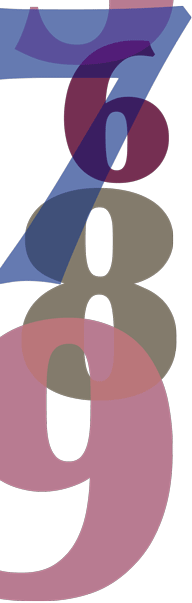# Year 6

## Number and Algebra

### FractionsDetailed description

A fraction has a number on top and a number down below. These numbers have special names. The top number is called the numerator. The number down below is called the denominator. The line between the numerator and the denominator is called the vinculum.

Equivalent fractions are equal even though they have different numerators and denominators. Equivalent fractions mark the same place on a number line.Detailed description

Equivalent fractions are produced by multiplying the numerator and the denominator by the same whole number. Equivalent fractions can also be produced by dividing the numerator and the denominator by the same whole number.

A facility with equivalent fractions helps students operate on them using addition, subtraction or multiplication. At this level we work mostly with fractions with related denominators. In simple cases, fractions are related if the denominator of one is a multiple of the other.